## research papersFOUNDATIONSADVANCES
ISSN: 2053-2733
Volume 61| Part 1| January 2005| Pages 139-146

## Determination of depth-dependent diffraction data: a new approach

aCentre for Materials Science and Engineering, Cranfield University, RMCS, Shrivenham, Swindon, Wilts SN6 8LA, England, and bApplied Mathematics and Operational Research Group, Cranfield University, RMCS, Shrivenham, Swindon, Wilts SN6 8LA, England

(Received 31 March 2004; accepted 22 October 2004)

A direct method for determining powder diffraction data at specific depths from angle-dependent diffraction data is described. The method is non-destructive and only traditional data collections, where the angle of incidence is varied, are required. These angle-dependent spectra are transformed to give diffraction data arising from different depths, which may then be exploited using any conventional method. This is a novel approach as traditional methods are forced to tolerate the inherent depth averaging of grazing-angle diffraction, or only examine specific structural characteristics. In order to obtain depth-dependent X-ray diffraction data, a Fredholm integral equation of the first kind is solved using regularization techniques. The method has been validated by the generation of pseudo-experimental data having known depth profiles and solving the Fredholm integral equation to recover the solution. The method has also been applied to experimental data from a number of thin film systems.

### 1. Introduction

The ability to characterize polycrystalline thin films with depth is of great interest in the field of materials science. Many current approaches to depth profiling are destructive, i.e. a small amount of material is removed from the uppermost layers systematically by methods such as gradual polishing or cutting (Singh & Low, 2002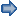). These methods may alter characteristics such as the stress state with each loss of material (Härting, 1998), and may also incorporate additional features such as etch pits and bevelling of the sample surface (Rogers et al., 1999). To interrogate samples in a non-destructive manner, the angle between the analysing probe and sample surface can be reduced, decreasing the effective depth of analysis. For any given incident angle, γ, the diffraction data obtained are an average of information from various depths over the depth of penetration, weighted towards the uppermost layers (Predecki, 1993; Luo et al., 1996; Wu et al., 2002). Until now, information from a specific depth below the surface of the sample could not be directly determined from the observed diffraction data (Wu et al., 2002). By transforming experimental data collected over a range of different incident angles, we aim to introduce a method of directly determining diffraction data arising from specific depths beneath the surface.

As with previous work (Wu et al., 2002; Li et al., 2000), our diffraction data will be recovered on an absorption depth scale. The work described here enables analysis of samples with discontinuities, a limitation in previous similar work (Li et al., 1999, 2000).

Predecki (1993) has previously described a method for determining profiles of quantities that are assumed functions of depth below the sample surface. Laplace transforms are used to perform this transformation and a known well defined function is fitted to the observed quantity profile and the solution then manipulated according to different cases (Luo & Tao, 1996). In contrast, our approach is a priori, making no assumptions about the form of the depth-dependent diffraction data.

This paper is an extension of our previous work (Broadhurst et al., 2004). We now apply Chebyshev polynomials to discretize the Fredholm integral equation instead of quadrature methods. This work will also present the transformation of experimental X-ray diffraction data into depth-dependent diffraction data on the scale of absorption depth and not the true depth, as any variations in the linear absorption coefficient with depth are not currently taken into account.

### 2. Method

#### 2.1. Experimental theory

For a particular angle of incidence, γ (see Fig. 1), the corresponding X-ray diffraction pattern measured in asymmetric parallel geometry is a weighted sum of the diffraction data from all depths up to the maximum penetration depth. For a sample with varying linear absorption coefficient with depth, the observed X-ray diffraction intensity may be determined from (Luo & Tao, 1996)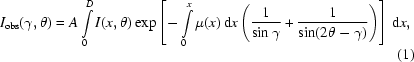where Iobs(γ,θ) = observed diffraction data collected from a sample, I(x,θ) = diffraction data from a specific depth, x, within the sample which we wish to determine, μ(x) = linear absorption coefficient of the material at depth x within the sample. The factor A takes into account the geometry of the system and also includes other factors such as refraction.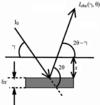Figure 1 Asymmetric Bragg geometry.

In this work, we do not consider the variation of μ with depth but solve in terms of the absorption depth X, where X =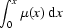and hence equation (1)may be rewritten as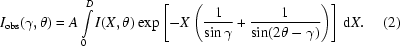The upper limit of this integral equation, D, is the maximum depth of penetration over which analysis takes place, and in this work is defined as the point at which the beam intensity has fallen by 95% of the intensity of the incident beam. θ appears only as a parameter and (2)needs to be solved at each θ considered.

For each θ, equation (1)has the form of a standard Fredholm integral equation of the first kind (Franken, 1997; Riele, 1985; Weese, 1993):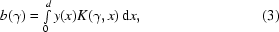where b(γ) is the scaled observed data, y(x) is the unknown solution to the integral equation that we wish to determine. K(γ,x) is the kernel and characterizes the physical process considered. The equation is solved at each θ value. This form of integral equation may be found in many other areas of science, such as the measurement techniques used in aerosol science (Franken, 1997), and is similar to topographic reconstructions in medical imaging (Curry et al., 1990). Fredholm integral equations of the first kind are ill conditioned (Baker et al., 1964) so small errors in b(γ) may cause large errors in y(x) (Hanna & Brown, 1991). Determination of y(x) is thus a mathematically ill-posed problem (Li et al., 1999; Wu et al., 2002).

Previously, it has been possible to modify equation (1)to accommodate samples with preferred orientation (Li et al., 1999, 2000).

#### 2.2. Solution method

In this work, we use numerical methods to find y at n discrete values of x: yi = y(xi) for i = 1, …, n.

In our previous work (Broadhurst et al., 2004), the integral equation (3)was discretized using Simpson's rule. Here, the integral equation has been discretized by representing the unknown yi as a sum of n Chebyshev polynomials,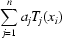. The collocation method is an improvement to quadrature as a smooth function is fitted to the solution points rather than a set of piecewise continuous functions. The aj are found using collocation to ensure that (3)is satisfied at each of the m values of γ for which the data has been collected.

Over the range [0, d], the Chebyshev polynomials are defined as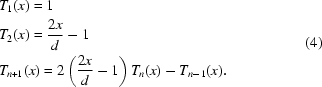The errors in the calculated yi can be minimized by choosing to collocate those values of x that correspond to the zeros of the nth Chebyshev polynomial, Tn. These are given by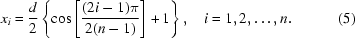Substituting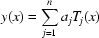into (3)yields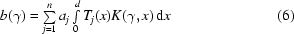at each of the m values of γ.

This can be rewritten as a matrix equation: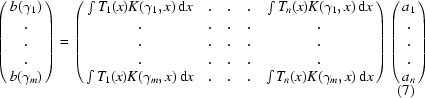or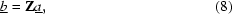where each of the matrix entries can be calculated as accurately as required using numerical quadrature.

The general case for this equation is when n ≠ m, and hence Z is not square and the system is defined as being either over- or under-specified. Equation (7)is thus solved using a least-squares method, with the aim of minimizing the square of the residual, r, of the solution, where: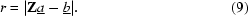It can be shown that |r2| is minimized at a given by the solution of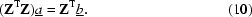Owing to the ill conditioned nature of the integral equation, this equation is also ill conditioned and is solved using regularization techniques. These attempt to remove the ill conditioning and allow meaningful solutions to be obtained.

##### 2.2.1. Regularization

Regularization techniques aid the solution of ill conditioned systems by the incorporation of additional assumed properties of the solution, such as smoothness. This is achieved by the addition of an extra term into the residual being minimized. When the solution exactly satisfies the assumed properties, this extra term reduces to zero. The residual to be minimized in this case is taken to be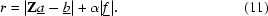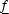is a function of the solution chosen to regularize the system and α is a weighting parameter. The weighting parameter α is chosen so that the solutions obtained are not over-smoothed but that the ill conditioning is sufficiently removed.

When using first-order regularization (constrained linear inversion), the regularizing function is chosen to minimize a measure of gradient of the solution y(x). Note that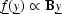and since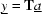,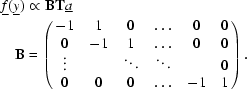For higher-order regularization, a different matrix B is used.

The value of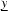that minimizes this equation can be shown to be given by the solution of the following equation.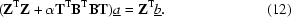Once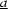has been determined, the solutionmay be easily obtained from the definition above.

### 3. Numerical method validation

In order to test the algorithm for determination of depth-dependent diffraction data, a number of systems were simulated, including a thick homogeneous sample, a thin homogeneous film, a bilayer sample and a sample with varying crystallite size with depth. For each system, pseudo-experimental data were generated for different combinations of m, the number of different incident angles for which simulated data were generated, and n, the number of terms in the collocating polynomial series. In each case, the spectra at each different incident angle was generated at 50 2θ values over an arbitrary 2θ range.

From numerical experiments performed, a value for α of 0.3 × tr(ZTZ)/tr(TTBTBT) was shown to remove the ill conditioning of the systems without `over-smoothing' the solutions obtained.

#### 3.1. Thick homogeneous sample

For this example, a single diffraction peak was considered in the 2θ range 32–38°, and it was assumed that at each depth the intensity of the diffraction peak remained constant with depth. Pseudo-experimental diffraction data were generated for 50 evenly spaced incident angles between 0.4 and 20° (Fig. 2a), where the minimum incident angle was above the critical angle for the system (μ = 454.2 cm−1, λ = 1.345 Å, γc ≃ 0.36°). Using the algorithm described previously, these angle-dependent pseudo-experimental data were transformed into the depth-dependent diffraction data using 50 terms in the collocating polynomial series (Fig. 2b). Here the r.m.s. error between the known and calculated solutions was found to be 0.066, where the maximum peak intensity was approximately 53.5. Fig. 2(c) is a plot of the differences between the calculated solutions and the model profile for a selection of 11 depths for which the solutions were determined.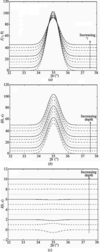Figure 2 (a) Generated pseudo-experimental data. (b) Recovered solutions after transformation. (c) Difference between calculated and assumed solution for a homogeneous sample. All spectra offset for clarity.

#### 3.2. Thin homogeneous film

Again, a single diffraction peak was considered over the 2θ range of 32–38° and this was used to model a film of polycrystalline material with a thickness less than that of the penetration depth of the X-rays at an incident angle of >1.8°. This model profile was used to generate simulated data at 50 evenly spaced incident angles over the same range as previously described (Fig. 3a). The depth-dependent solution using 50 terms in the collocating polynomial series was then calculated (Fig. 3b), and in this case the r.m.s. error was determined as being 1.729, with a maximum peak intensity of approximately 56.7. Fig. 3(c) shows the intensities of the known and calculated solutions for the peak maximum. As expected, the higher errors compared to the previous example arise from the fact that a discontinuity is present in the assumed solution. However, the use of Chebyshev polynomials has improved the form of the recovered solution over the use of Simpson's rule in our previous work (Broadhurst et al., 2004). It is noted that there are oscillations around zero in the region where no peak exists in the known solution. The use of a non-negativity constraint to prevent this behaviour will be considered in future work.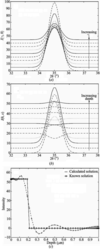Figure 3 Thin homogeneous film. (a) Generated pseudo-experimental data. (b) Recovered solutions after transformation. Spectra offset for clarity. (c) Calculated and known intensity of peak maximum with depth.

The errors in this instance can be seen to arise around the discontinuity, i.e. the back edge of the film. The maximum errors in this case are determined by the intensity of the peak. In addition, it can be seen that the errors in the regions where no peak is present are relatively small in relation to the errors around the discontinuity.

#### 3.3. Bilayer

Diffraction data from a bilayer system were simulated using materials giving diffraction peaks in the range of 31–37°. For this material system, evenly spaced incident angles between 0.4 and 20° were again considered, due to the critical angle being approximately 0.36° for an incident X-ray wavelength of 1.345 Å, but the 2θ range was altered to be between 30 and 38°. The peak due to the upper layer was present in the range of 31–33° and was present until an arbitrary depth, relating to an incident angle of 3.8°, from which depth onwards the peak due to the lower layer, observed in the range of 35–37°, was present. Again, simulated data were generated for 50 incident angles (Fig. 4a), and these were then transformed into depth-dependent data using 50 terms in the collocating polynomial series (Fig. 4b). The r.m.s. error was determined to be 4.434, where the intensity of the Bragg peak from the upper layer was approximately 105 and the maximum intensity of the Bragg peak of the lower layer was approximately 240. As with the thin homogeneous film sample, the errors are higher than for the homogeneous thick sample system, and this is again due to the discontinuities present in the model profile, around which the maximum errors are observed. Again, in the region where no peaks are present, the errors are relatively small. Fig. 4(c) shows the intensities of the known and calculated solutions for the peak maxima with depth.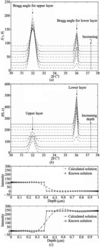Figure 4 Bilayer. (a) Generated pseudo-experimental data. (b) Recovered solutions after transformation. Spectra offset for clarity. (c) Calculated and known intensity of peak maxima with depth.

#### 3.4. Varying crystallite size

For this material system, the depth-dependent data were assumed to vary in a continuous manner with the crystallite size varying from large to small with increasing depth. This gives rise to a peak, in the region of 32–38°, which broadens with depth. Simulated data were generated with 50 evenly spaced incident angles over the range 0.4–20° (Fig. 5a), and this was transformed into depth-dependent data using the solving algorithm with 50 terms in the collocating polynomial series (Fig. 5b). The r.m.s. error associated with this calculated solution was determined as 1.616, with a peak intensity varying from approximately 103 to 36.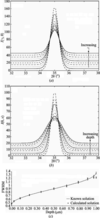Figure 5 (a) Generated pseudo-experimental data. (b) Recovered solutions after transformation. Spectra offset for clarity. (c) FWHM versus depth for sample with varying crystallite size.

In this instance, errors arise as the shape of the calculated solution does not exactly match that of the model profile in that the intensity does not drop as sharply as the model profile in the uppermost layers. However, the peak widths are mapped well in comparison to the model profile, as identified in Fig. 5(c), which shows the FWHM of both the model profile and the calculated solutions with their associated errors.

The above generations of simulated data and their subsequent transformations to depth-dependent data for a range of samples show that depth-dependent diffraction data can be directly determined from data collected at a range of different incident angles for a range of materials systems.

### 4. Application to experimental data

Asymmetric parallel-beam diffraction data were collected from (i) a thin film of copper on glass and (ii) a thin electrodeposited hydroxyapatite layer on titanium. These data were collected using station 2.3 at the Synchrotron Radiation Source (SRS), Daresbury, and have to be pre-processed before being used in the solution algorithm described.

In order for the incident X-ray beam not to overspill the sample, the area of the beam on the sample was varied for each incident angle. This geometric correction was applied to the intensity measurements before transformation of the data was performed.

The effect of refraction on the diffraction data was also considered before transformation of the angle-dependent diffraction data. This correction has been described elsewhere (Lim et al., 1987; Toney et al., 1988; Neerinck & Vink, 1996; Rigden et al., 1997; Kim et al., 2003).

#### 4.1. Copper on glass

The nominal structure of this sample was a 300 nm thin film of copper on a glass substrate.

The range of incident angles used for analysis of this sample was 0.5–5° in 0.5° steps, resulting in ten different angles of incidence (the critical angle for copper being approximately 0.36°). The count time was 1 s per point, and the data were collected over a 2θ range of 36 and 46° in step sizes of 0.01°.

Fig. 6shows the data collected after all corrections for geometry, refraction and beam sizes have been applied. Using the solving algorithm described, these data were then transformed into depth-dependent data (Fig. 7) using 16 terms in the collocating polynomial series.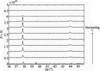Figure 6 Data collected for copper on glass; corrected for refraction, beam widths and geometry.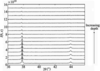Figure 7 Depth-dependent diffraction data for copper on glass.

The errors in the intensity of the observed pattern are dependent on the time taken to accumulate the pattern. In order to investigate this, the error in the count rate for this sample will be determined by calculating the total counts accumulated over a selected angular range where a peak exists on the diffraction pattern, Np, and the associated counts due to the background, Nb, over this angular range (Jenkins & Snyder, 1996).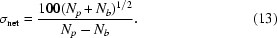The percentage error in the signal has been calculated as being approximately 1.1%.

#### 4.2. Electrodeposited hydroxyapatite sample

Calcium hydroxyapatite (HAP) is a material that can be used in bone defect reconstruction (Schnettler et al., 2003). It may also be used for coating bioprostheses such as hip replacements, as it is a biocompatible and bioactive ceramic (Sridhar et al., 2003) that provides an interface between the metallic substrate and the tissue surrounding the implant, providing an improvement in the integration into the bone (Souto et al., 2003).

Diffraction data were collected from an electrodeposited sample of HAP on a titanium substrate. For incident angles between 0.35 and 0.8°, a step size of 0.05° was used, which was increased to 0.1° for angles of incidence between 0.9 and 2.7° (critical angle for HAP is approximately 0.22°). This gives 29 observed data patterns collected over a 2θ range of 21.5 to 35.5° with a step size in the 2θ direction of 0.01°, and a count time of 1.2 s per point for incident angles up to 1.8°. This was decreased to 1.1 s per point for all remaining incident angles. The percentage error in the observed intensity was calculated as being approximately 1%.

Using the corrections for refraction, varying beam widths used and geometrical factors, the diffraction data for HAP collected at the previously defined incident angles (Fig. 8) were transformed from angle-dependent data to depth-dependent data using the solving algorithm previously outlined with 29 terms in the collocating polynomial series (Fig. 9).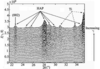Figure 8 Data collected for electrodeposited HAP on Ti; corrected for refraction, beam widths and geometry.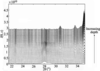Figure 9 Depth-dependent diffraction data for electrodeposited HAP on Ti.

### 5. Conclusions

A method for directly determining the X-ray diffraction data at specific depths has been presented. The method was validated by recovering depth profiles from simulated data. The method was also applied to data collected in diffraction experiments in which the incident angle was varied and a number of corrections applied.

In this study, the diffractograms obtained with depth have been presented on the absorption depth scale. If, however, the linear absorption coefficient were constant throughout the entire depth of penetration of the incident X-ray beam, then the depth scale would become a direct depth scale.

The results obtained for the thin film of copper on a glass substrate indicate that the thickness of the copper layer is approximately 317 nm determined by identifying the point where the intensity had fallen to half that of the copper peaks. The precise depth cannot be determined due to errors in the solving process outlined previously for a sample containing a discontinuity, as in this instance, and also because a solution is not determined for the infinite number of depth values.

The transformed data for the HAP on titanium sample indicates that the thickness of the HAP layer is approximately 1.82 µm. As can be seen, there is an increase in the noise at greater depths. This is due to the propagation of errors incurred from the uppermost layers, and may also be because more information is obtained for the uppermost layers than those towards the greater depths as information for the uppermost layers is obtained at all incident angles, whereas information about the lower layers will only be obtained at the highest incident angles. From this depth-dependent data, the FWHM of the 002 peak appears broader at the uppermost layers, indicating a decrease in the crystallite size at the uppermost layers of the sample; this can clearly be seen in Fig. 10.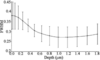Figure 10 FWHM with depth of the (002) peak for HAP.

Systematic errors within the experimental data will be unaffected by the regularization processes and the transformed depth-dependent data will still contain these errors. Fluctuations in the transformed depth-dependent data due to errors in the collected experimental data are reduced or damped by the regularization process as the ill conditioning that this induces is removed. We are currently investigating the influence that these errors have in the depth-dependent data. For example, for a bilayer system and 10% random error in the data, the errors between the depth-dependent solution and the known model profile have been calculated as being 116.7 (maximum error) and 9.9 (r.m.s. error); hence, reasonable results may still be obtained with this level of error in the experimental data. Where errors in the data are present, more regularization needs to be included into the system for solution as opposed to the model with no errors in the generated data.

In addition to regularization in the x direction as outlined in this study, trials using regularization in the 2θ direction using pseudo-experimental data have been attempted. However, the solutions obtained have been poorer with this additional regularization than without. Hence, the work outlined here does not include this additional regularization in the solving algorithm for both pseudo-experimental and real experimental data.

The technique outlined in this work is novel as it is diffractograms from discrete depths that are recovered rather than depth profiles of specific characteristics. Thus, a variety of structural characteristics can subsequently be obtained at specific depths by applying conventional diffraction tech­niques, such as Rietveld analysis.

The method described in this work is being enhanced to include changes to the linear absorption coefficient with depth, which will allow diffractograms on a direct depth scale to be determined. The revised algorithm will then be applied to data collected from a range of samples on both the synchrotron at Daresbury and also on laboratory-based equipment.

Our analytical process may be applied generically to any experimental technique where the depth of analysis is reduced when the angle of incidence between the sample surface and the incident analysing probe is reduced. We are currently assessing the method for particle induced X-ray emission (PIXE), which may be used to find information about trace elements present in a sample at various depths. In this case, the kernel of the Fredholm integral equation will be altered to characterize the processes taking place in PIXE, and data will be collected and transformed from angle-dependent data to depth-dependent data for this analytical technique.

### Acknowledgements

This work is made possible by an Engineering and Physical Sciences Research Council (EPSRC) project grant (GR/R19700).

### ReferencesFOUNDATIONSADVANCES